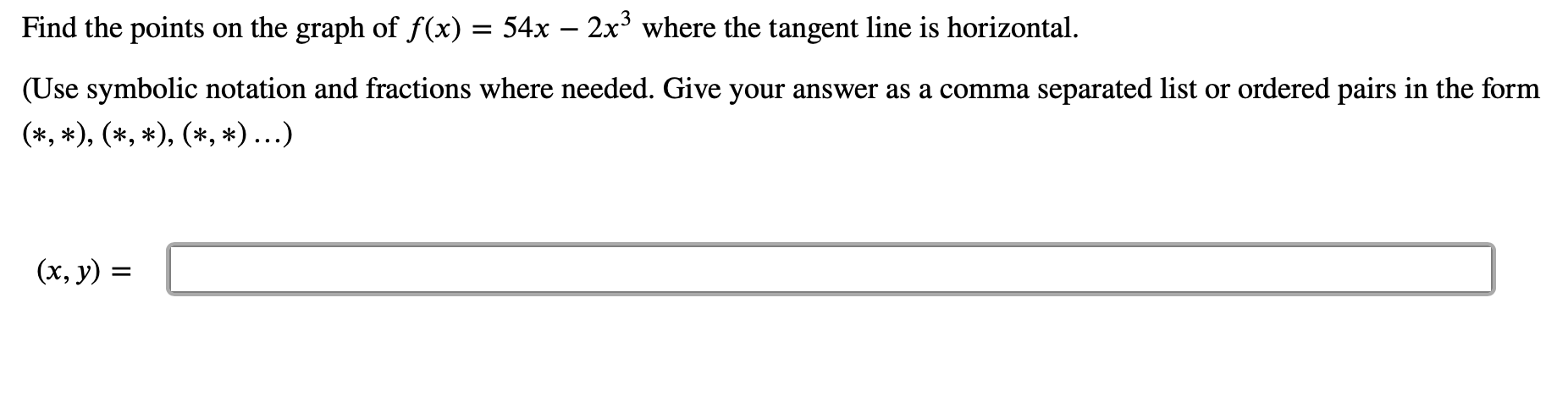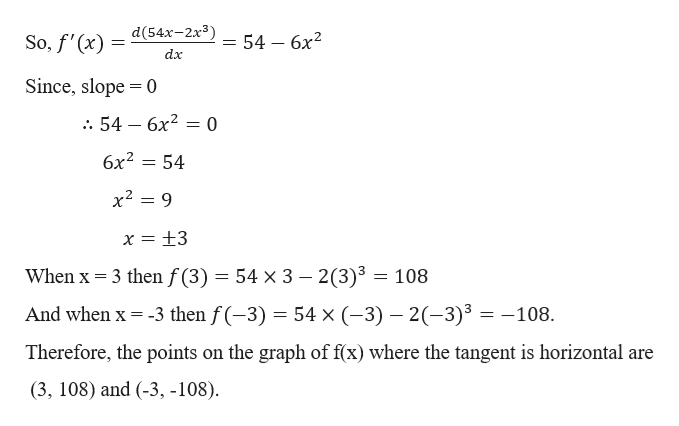# on the graph of f(x) = 54x - 2x3 where the tangent line is horizontalFind the points(Use symbolic notation and fractions where needed. Give your answer as a comma separated list or ordered pairs in the form(*, *), (*,*(, *)...)(x, y)

Question
1 viewshelp_outlineImage Transcriptioncloseon the graph of f(x) = 54x - 2x3 where the tangent line is horizontal Find the points (Use symbolic notation and fractions where needed. Give your answer as a comma separated list or ordered pairs in the form (*, *), (*,* (, *)...) (x, y) fullscreen
check_circle

Step 1

Given,

Step 2

Here we have to find the points on the graph of f(x) where the tangent is...help_outlineImage TranscriptioncloseSo,f'(x d(54x-2x3) dx 54 — бх2 Since, slope 0 : 54 — бх2 — 0 11 6x2 54 x2 9 When x 3 then f (3) 54 x 3 - 2(3)3 = 108 And when x -3 then f(-3) 54 x (-3) 2-3)3 108 Therefore, the points on the graph of f(x) where the tangent is horizontal are (3, 108) and (-3, -108) fullscreen

### Want to see the full answer?

See Solution

#### Want to see this answer and more?

Solutions are written by subject experts who are available 24/7. Questions are typically answered within 1 hour.*

See Solution
*Response times may vary by subject and question.
Tagged in

### Derivative